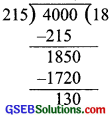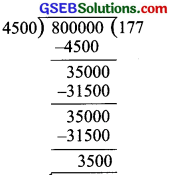# GSEB Solutions Class 6 Maths Chapter 1 Knowing Our Numbers Ex 1.2

Gujarat Board GSEB Textbook Solutions Class 6 Maths Chapter 1 Knowing Our Numbers Ex 1.2 Textbook Questions and Answers.

## Gujarat Board Textbook Solutions Class 6 Maths Chapter 1 Knowing Our Numbers Ex 1.2

Question 1.
A book exhibition was held for four days in a school. The number of tickets sold at the counter on the first, second, third and final day was respectively 1094, 1812, 2050 and 2751. Find the total number of tickets sold on all the four days.
Solution:
Number of tickets sold on
The first day = 1094
The second day = 1812
The third day = 2050
The fourth day = 2751
∴ Total number of tickets sold on all the four
days 1094 + 1812 + 2050 + 2751 = 7707.

Question 2.
Shekhar is a famous cricket player He has so far scored 6,980 runs in test matches. He wishes to complete 10,000 runs. How many more runs does he need?
Solution:
Number of runs scored by Shekhar so far = 6,980
Target of runs to be scored = 10,000
∴ Number of runs needed more = 10,000 – 6,980 = 3,020Question 3.
In an election, the successful candidate registered 5,77,500 votes and his nearest rival secured 3,48,700 votes. By what margin did the successful candidate win the election?
Solution:
∴Number of votes secured by the successful candidate = 5,77,500
Number of votes secured by his rival = 3,48,700
Margin of votes = 5,77,500 – 3,48,700
= 2,28,800

Question 4.
Kirjj bookstore sold books worth 2,85,891 in the first week of June and books worth 4,00, 768 in the second week of the month. flow much was the sale for the two weeks together? In which week was the sale greater and by how much?
Solution:
Sale of books in
1 st week = ₹ 2,85,891
2nd week = ₹ 4,00,768
Total sale for the two weeks together
= ₹ 2,85,89 1 + ₹ 4,00,768 = ₹ 6,86,659
Obviously, the sale was greater in the second week by ₹ 4,00,768 – ₹ 2,85,89 1
i.e. by ₹ 1,14,877.Question 5.
Find the difference between the greatest and the least number that can be written using the digits 6, 2, 7, 4, 3 each only once.
Solution:
Given digits are 6, 2, 7, 4 and 3.
Greatest number made by using these digits = 76,432
Smallest number made by using these digits = 23,467
∴Difference = [greatest number] – [smallest number]
= 76,432 – 23,467 = 52,965

Question 6.
A machine, on an average, manufactures 2,825 screws a day. How many screws did it produce in the month of January 2006?
Solution:
Number of days in January 2006 = 31
Number of screws manufactured in one day = 2,825
∴ Number of screws manufactured in 31 days = 31 x 2,825 = 87,575
∴ Number of screws manufactured in the month of January 2006 = 87,575.Question 7.
A merchant had ₹ 78,592 with her. She placed an order for purchasing 40 radio sets at ₹ 1200 each. How much money will remain with her after the purchase?
Solution:
Number of radio sets to be purchased = 40
Cost of one radio set = ₹ 1200
Cost of 40 radio sets = 40 x ₹ 1200
= ₹ 48,000
Total money with the merchant = 78,592
Money left with the merchant after the purchase of 40 radio sets
= ₹ 78,592 – ₹ 48,000 = ₹ 30,592

Question 8.
A student multiplied 7236 by 65 instead of multiplying by 56. By how much was his answer greater than the correct answer?
Hint: Do you need to do both the multiplications?
Solution:
The required multiplication = 7236 x 56
The multiplication done by the student = 7236 x 65
Difference = [7236 x 65] – [7236 x 56]
= 7236 [65 – 56]
= 7236 x 9 = 65124Question 9.
To stitch a shirt, 2 m 15 cm cloth is needed. Out of 40 m cloth, how many shirts can be stitched and how much cloth will remain?
Hint: Convert data in cm.
Solution:
Cloth required to stitch 1 shirt
= 2m 15 cm
= 200 cm + 15 cm [ 1 m = 100 cm]
= 215cm
Total cloth = 40 m
= 40 x 100 cm
= 4000 cm
SinceNumber of shirts = 4000 cm ÷ 215 cm
= 18 shirts + 130 cm
Thus, 18 shirts can be stitched and cloth leftover is 1 m 30 cm.Question 10.
Medicine is packed in boxes, each weighing 4 kg 500 g. How many such boxes can be loaded in a van which cannot carry beyond 800 kg?
Solution:
Weight of 1 box = 4 500 g
= 4000 g + 500 g [ 1 kg = 1000 g]
= 4500 g
Maximum weight which can be loaded = 800 kg
= 800 x 1000 g = 800000 g
No. of boxes = 800000 g ÷ 4500 g
= 177 x 4500 g + 3500 g
Since∴ 177 boxes can be loaded in the van.Question 11.
The distance between the school and the house of a student is 1 km 875 m. Every day she walks both ways. Find the total distance covered by her in six days.
Solution:
Distance between school and house
= 1 km 875 m
= 1000 m + 875 m [ 1 km = 1000 m]
= 1875 m
∴ Distance covered by the student both ways
between the school and house in one day = 2 x 1875 m = 3750 m
Thus, distance covered in 6 days = 6 x 3750 m
= 22500 m = 22 km 500 m

Question 12.
A vessel has 4 liters and 500 ml of curd. In how many glasses, each of 25 ml capacity, can it be filled?
Solution:
Since, 4 litres 500 ml= (4 x 1000) ml + 500 ml [ 1 l = 1000 ml]
= 4000 ml + 500 ml = 4500 ml
Capacity of 1 glass = 25 ml
∴Number of glasses that can be filled
= 4500 ml ÷ 25 ml = 180Thus, 180 glasses can be filled.